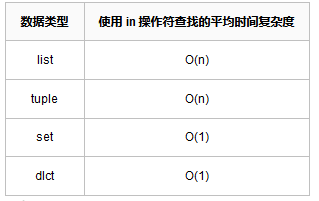### 前言

Python中 in 操作符可以用于判断某个元素是否存在于当前对象中，而对于不同的Python对象，使用 in 操作符的处理效率是不一样的。

### 测试过程

``````import time
import random

def demo(target, num):
time1 = time.time()
res = []
for i in range(num):
if i not in target:
res.append(i)
time2 = time.time()
print("结果：{}，当前类型：{}，耗时：{}".format(res, type(target), time2 - time1))

num = 500
tmp_set = set()
while len(tmp_set) <= num - 3:
tmp_list = list(tmp_set)
tmp_tuple = tuple(tmp_set)
tmp_dict = {key: "" for key in tmp_set}

demo(tmp_list, num)
demo(tmp_tuple, num)
demo(tmp_set, num)
demo(tmp_dict, num)
``````

``````不包含的整数：[25, 31, 36]，当前类型：<class 'list'>，耗时：0.0

``````

``````不包含的整数：[114, 329, 355]，当前类型：<class 'list'>，耗时：0.0059354305267333984

``````

``````不包含的整数：[445, 850, 3547]，当前类型：<class 'list'>，耗时：0.3342933654785156

``````

``````不包含的整数：[9296, 18652, 32281]，当前类型：<class 'list'>，耗时：26.92029118537903

``````

``````不包含的整数：[16086, 33891, 46161]，当前类型：<class 'list'>，耗时：52.91718029975891

``````

``````不包含的整数：[23057, 35827, 69232]，当前类型：<class 'list'>，耗时：75.57932734489441

``````

``````'''

'''

``````

### 结论

list列表、tuple元组、set集合、dict字典，使用 in 操作符查找的平均时间复杂度如下：